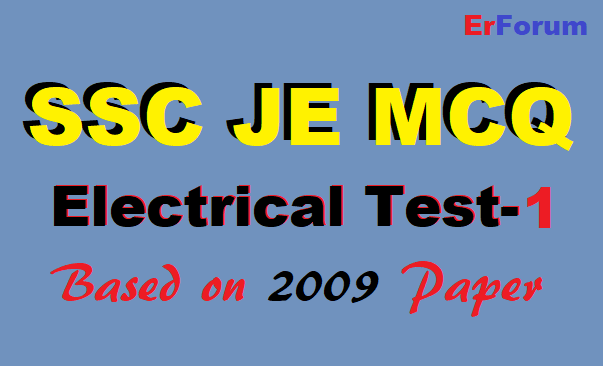3/recent/ticker-posts

# SSC JE Electrical 2009 MCQ Test-1

## SSC JE Electrical 2009 MCQ Test-1

This MCQ Test is based on SCC JE 2009 official Paper.1. Earth fault relays are:
(A) Directional relays
(B) Non-directional relays
(C) Short operate-time relays

(D) Long operate-time relays

2. The rating of the fuse is expressed in terms of:
(A) amperes
(B) volts
(C) VAR
(D) KVA

3. By burden of the relay we mean:
(A) volt-ampere rating of the relay
(B) the current rating of the relay
(C) the voltage rating of the relay
(D) watt rating of the relay

4. Reactance relays are employed for phase fault in:
(A) long time
(B) medium line
(C) short line
(D) any of these

5. The ratio of line-to-line capacitance and line-to-neutral capacitance is:
(A)1/2
(B)1/4
(C) 2
(D) 4

6. The material commonly used for sheaths of underground cable is:
(B) steel
(C) rubber
(D) copper

7. The recovery voltage will be maximum for a power factor of:
(A) zero
(B) 0.5
(C) 0.707
(D) unity

8. An air blast circuit breaker is usually employed for:
(A) instantaneous voltage
(B) intermittent duty
(C) repeated duty
(D) short duty

9. The value of current I flowing through the 1 Ω resistor in the circuit shown in the figure below will be:(A) 10A
(B) 5A
(C) 6A
(D) Zero

10. In the figure shown below if we connect a source of 2V, with an internal resistance of 1Ω at AA’ with internal at A, the current through R is:(A) 2 A
(B) 1.66 A
(C) 1 A
(D) 0.625 A

11. The curve representing Ohm’s law is:
(A) Linear
(B) Hyperbolic
(C) Parabolic
(D) Triangular

12. The Specific resistance of a conductor depends upon:
(A) The dimension of the conductor
(B) Composition of conductor
(C) The resistance of the conductor
(D) Both (A) and (B)

13. Superposition theorem is essentially based on the concept of:
(A) Reciprocity
(B) Linearity
(C) Duality
(D) Non-linearity

14. If a 500 KVA, 200 Hz transformer is operated at 50 Hz, its KVA rating will be:
(A) 2000 KVA
(B) 125 KVA
(C) 250 KVA
(D) 1000 KVA

15. The angle between induced emf and the terminal voltage on no-load for a single phase alternator is:
(A) 180°
(B) 90°
(C) 0°
(D) 270°

16. A salient pole synchronous generator connected to an infinite bus power will deliver maximum power at a power angle of :
(A) δ=0°
(B) δ=90°
(C) δ=45°
(D)δ=30°

17. Starting torque of the synchronous motor is :
(A) very low
(B) zero
(C) very high

18. The power factor at which transformer operates:
(A) is unity
(B) is 0.8 lag
(D) depends upon the power factor of the load

19. The efficiency of a 100 KVA transformer is 0.98 at full as well as half load for this transformer at full load the copper loss :
(A) is less than core loss
(B) is equal to core loss
(C) is more than core loss
(D) All the above

20. Which of the following will improve the mutual coupling between primary and secondary circuit?
(A) Transformer oil of high breakdown voltage
(B) High reluctance magnetic core
(C) Winding material of high resistivity
(D) Low reluctance magnetic core

21. High leakage transformers are of:
(A) small voltage ampere rating
(B) high voltage ampere rating
(C) low voltage rating
(D) low voltage rating

22. The starting torque of a 3-phase induction motor varies as :
(A) V2
(B) V
(C)
(D)1/V

23. In a 3-phase induction motor, the mechanical power developed; in terms of air gap power Pg is :
(A) (1-S)Pg
(B) PgS
(C) Pg /(1-S)
(D) Pg /S

24. The negative phase sequence in a 3-phase synchronous motor exists when the motor is :
(C) Supplied with unbalanced voltage
(D) Hot

25. A centre zero ammeter connected in a rotor circle of a 6 pole, 50 Hz induction motor makes 30 oscillations in one minute. The rotor speed is:
(A) 670 rpm
(B) 990 rpm
(C) 1010 rpm
(D) 1030 rpm

MCQ 26-38: Check here

SSC JE Electrical 2004 to 2016 Official/Original Previous Papers- Find here